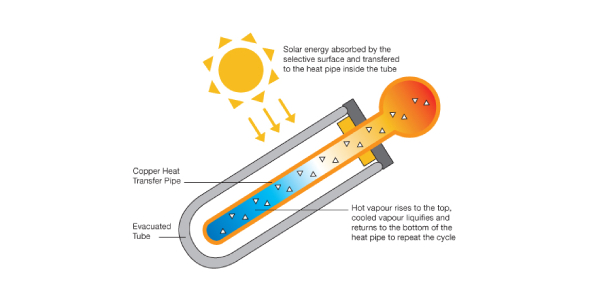# Thermal Energy Trivia: Ultimate Knowledge! Quiz

8 Questions | Total Attempts: 1575SettingsWhat do you know about geothermal energy? Geothermal energy produces one-sixth of the carbon dioxide produced by a natural gas plant and is not an intermittent source of energy like wind or solar. Do you think this type of energy is beneficial or is accessing it dangerous? This quiz is made up of ultimate trivia questions designed to teach you some more about this source of energy, give it a shot!

• 1.
The thermal energy that flows from something at a higher temperature to something at a lower temperature. Name which definition this is matches to.
• A.

Conduction

• B.

• C.

Heat

• D.

Specific heat

• E.

None of the above

• 2.
The sum of kinetic and potential energy of all the particles in an object. Name which definition this matches to.
• A.

Temperature

• B.

Heat

• C.

Conduction

• D.

Insulator

• E.

None of the above

• 3.
What is the Equation for Thermal Energy ( also known as the Thermal Energy equation)?
• A.

There is no equation.

• B.

Volume+mass x length

• C.

Mass x change in temperature x specific heat

• D.

Length x width x height

• E.

3.14 x 2r

• 4.
____________ is the measure f the average kinetic energy of the particles in the object.
• 5.
____________ is the transfer of thermal energy by collisions between particles in the matter ( this occurs because particles in the matter are constantly moving).
• 6.
The amount of heat that is needed to raise the temperature of 1kg of some material by 1 degree Celsius. Match this definition with its word.
• A.

Specific heat

• B.

I don't know

• C.

Convection

• D.

Thermodynamics

• E.

Solar Collectors

• 7.
An active solar heating system that absorbs radiant energy from the sun. Which word does this definition belong to?
• A.

Thermodynamics

• B.

Solar collectors

• C.

Specific Heat

• D.Back to top